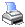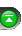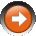﻿ Standard Deviation (StdDev)
 NinjaScript > Language Reference > Indicator Methods > Standard Deviation (StdDev)Description

In probability theory and statistics, standard deviation is a measure of the variability or dispersion of a population, a data set, or a probability distribution. A low standard deviation indicates that the data points tend to be very close to the same value (the mean), while high standard deviation indicates that the data are “spread out” over a large range of values.

... Courtesy of Wikipedia

Syntax

 StdDev(int period) StdDev(IDataSeries input, int period)   Returns default value StdDev(int period)[int barsAgo] StdDev(IDataSeries input, int period)[int barsAgo]

Return Value

double; Accessing this method via an index value [int barsAgo] returns the indicator value of the referenced bar.

Parameters

 input Indicator source data (?) period Number of bars used in the calculation

Examples

 // Prints the current value of a 20 period StdDev using default price type double value = StdDev(20); Print("The current StdDev value is " + value.ToString());   // Prints the current value of a 20 period StdDev using high price type double value = StdDev(High, 20); Print("The current StdDev value is " + value.ToString());

Source Code

You can view this indicator method source code by selecting the menu Tools > Edit NinjaScript > Indicator within the NinjaTrader Control Center window.